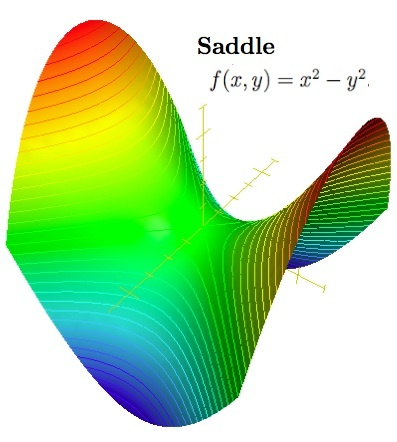# Hessian矩阵与多元函数极值

• 多元函数极值问题
• 泰勒展开式与Hessian矩阵

## 多元函数极值问题

fx=0fy=0fx=0

H=2fxx2fyx2fzx2fxy2fyy2fzy2fxz2fyz2fzz

H=2fx212fx2x12fxnx12fx1x22fx222fxnx22fx1xn2fx2xn2fx2n• 如果是正定矩阵，则临界点处是一个局部极小值
• 如果是负定矩阵，则临界点处是一个局部极大值
• 如果是不定矩阵，则临界点处不是极值

f(x1,x2,,xn)=a11x21+a22x22++annx2n+2a12x1x2+2a13x1x3++2an1,nxn1xn

f==a11x21+a12x1x2++a1nx1xn+a21x2x1+a22x22++a2nx2xn++an1xnx1+an2xnx2++annx2ni,j=1naijxixj

f===x1(a11x1+a12x2++a1nxn)+x2(a21x1+a22x2++a2nxn)++xn(an1x1+an2x2++annxn)(x1,x2,,xn)a11x1+a12x2++a1nxna21x1+a22x2++a2nxnan1x1+an2x2++annxn(x1,x2,,xn)a11a21an1a12a22an2a1na2nannx1x2xn

A=a11a21an1a12a22an2a1na2nann,x=x1x2xn

## 泰勒展开式与Hessian矩阵

f(x)=f(x0)+f(x0)(xx0)+f(x0)2!(xx0)2++f(n)(x0)n!(xx0)n+Rn(x)

Rn(x)=f(n+1)(ξ)(n+1)!(xx0)n+1

f(x,y)=f(x0,y0)+[(xx0)x+(yy0)y]f(x0,y0)+12![(xx0)x+(yy0)y]2f(x0,y0)+++1n![(xx0)x+(yy0)y]nf(x0,y0)+1(n+1)![(xx0)x+(yy0)y](n+1)f[x0+θ(xx0),y0+θ(yy0)]

[(xx0)x+(yy0)y]f(x0,y0)

(xx0)fx(x0,y0)+(yy0)fy(x0,y0)

[(xx0)x+(yy0)y]2f(x0,y0)

(xx0)2fxx(x0,y0)+2(xx0)(yy0)fxy(x0,y0)+(yy0)2fyy(x0,y0)

[(xx0)x+(yy0)y]mf(x0,y0)

p=0mCpm(xx0)p(yy0)(mp)mfxpy(mp)(x0,y0)

f(x,y)=k=0n1k![(xx0)x+(yy0)y]kf(x0,y0)+1(n+1)![(xx0)x+(yy0)y](n+1)f[x0+θ(xx0),y0+θ(yy0)],(0<θ<1)

f(x,y)=k=0n1k![(xx0)x+(yy0)y]kf(x0,y0)+o(ρn)

f(X)=f(X0)+(XX0)Tf(X0)+12!(XX0)T2f(X0)(XX0)+o(XX02)

f(X)=f(X0)+(XX0)Tf(X0)+12(XX0)TH(X0)(XX0)+o(XX02)

f(M)={fx1,fx2,,fxn}M=0